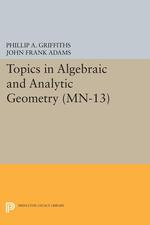# Topics in Algebraic and Analytic Geometry. (MN-13): Notes From a Course of Phillip Griffiths

PHILLIP GRIFFITHS
Written and revised by JOHN ADAMS
Pages: 228
https://www.jstor.org/stable/j.ctt130hk5x

1. Front Matter
(pp. i-iv)
(pp. v-vi)
3. Chapter One
(pp. 1-22)

On ₵n, the space of n complex variables, there is a sequence of sheaves defined in the usual topology:

\mathrm{O_{cont}}\supset \mathrm{O_{diff}}\supset \mathrm{O_{hol}}\supset \mathrm{O_{alg}}

where for an open set U⊂₵n:

Γ(U, Ocont)= continuous complex-valued functions defined on U.

Γ(U, Odiff)= Ccomplex-valued functions defined on U.

Γ(U, Ohol)= holomorphic functions defined on U.

Γ(U, Oalg)- rational holomorphic functions defined on U.

(A holomorphic function φ on U is said to be rational just in case each a in U has a neighborhood W in U such that there are two polynomials p,q with q nowhere zero in W, with φ-p/q in W. In Fact,...

4. Chapter Two
(pp. 23-55)

We shall deal extensively with sheaves of modules on a ringed space. Our treatment of basic definitions will be cursory and sometimes incomplete. For more details, see Godement .

What one means by an O - module on the ringed space (x,O) is a shealf of abelian groups on x such that the sections over any open set are a module over the sections of O on that open, and such that the module structures are compatible with the restriction maps. We have already seen some examples of sheaves of modules in sheaves of ideals.

Some constructions dealing with sheaves...

5. Chapter Three
(pp. 56-72)

Some basic facts in complex function theory are entirely local in character and these go over directly to the situation of a complex manifold. Often revised versions will hold for analytic spaces. One might expect that the way to generalize would be to resolve singularities, which is always possible. But it is easier to use the technique already mentioned in Chapter One of exhibiting an analytic space as locally a branched covering of a polydisk in ₵n. We now state the basic fact formally:

III. A. THEOREM If x is a point on an analytic space X there is a...

6. Chapter Four
(pp. 73-82)

In this chapter we shall prove comparison theorems about sheaves and sheaf cohomology. The groundwork for this has already been laid in the computation of the holomorphic and algebraic cohomology of the sheaves OPn(d) on projective space.

If X is a projective algebraic variety we shall sometimes use the notations Xalgfor X as a ringed space in the Zariski topology with sheaf Oalg, and Xholfor X as an analytic space. There is a morphism of ringed spaces

φ: Xhol→Xalg

We know from sheaf theory that any coherent algebraic sheaf F will pull back to a coherent analytic sheaf...

7. CHAPTER FIVE
(pp. 83-135)

Every complex manifold has a natural orientation, so that on a compact complex manifold M of dimension n there i s defined a prefered generator of H2n(M, Z)

If D is a complex submanifold of codimension one of the compact complex manifold M then the image of the prefered generator of H2n-2(D, Z) defines a class [D] in H2n-2(M, Z) and by Poincare duality a class [D] in H²(M, Z). This is the cohomology class of the divisor.

If M is a possibly non-compact complex manifold and D is a divisor, possibly with singularities, we can still define [D], ∈...

8. Chapter Six
(pp. 136-153)

The results in this chapter will be purely topological. Our topological spaces will always be paracompact, locally compact Hausdorff spaces, having the homotopy type of a finite simplicial complex; we shall call such spaces nice spaces. To each nice space X we will associate a ring K(X), which will classify the complex vector bundles on X up to a geometrically describable equivalence relation.

The Bott periodicity theorem gives an isomorphism

\mathrm{K(X)\otimes K(P^{1})\widetilde{\rightarrow }K(X)\times P^{1}}

This isomorphism will be the essential ingredient in the construction of a natural isomorphism

\mathrm{K(X)\otimes Q\widetilde{\rightarrow }H^{.,even}(X,Q)}

which will show that, over Q, the even-dimensional cohomology classes are combinations of the...

9. Chapter Seven
(pp. 154-195)

The goal of this chapter is to compare K-theory and ordinary cohomology. We shall give two different proofs of the basic theorem, one directly involving obstruction theory and the other a more formal proof involving a spectral sequence. At the end of this chapter we shall apply our results to study algebraic cocycles on a projective variety.

We shall study the Chern character, a map from Vect (X) H.,even(X,Q) which factors through K(X). The Chern class map from Vect (X) to H.,even (X,Q)will not factor through K(X) because it is not additive. The Chern character, on the other hand,...

10. Chapter Eight
(pp. 196-214)

This chapter continues the study of vector bundles, now from an analytic point of view. Our results will tend to compare what can be done continuously on a complex manifold with what can be done holomorphically. For this purpose some study of Stein manifolds will be necessary.

A Stein manifold is a complex manifold with a strictly plurisubharmonic exhaustion function; that is, a complex manifold M will be Stein just in case there is a function τ:M→R+, which is C², such that τ-1([0, ɑ]) is compact for all ɑ > 0, and τ is strictly plurisubharmonic. This means that in...

11. Chapter Nine
(pp. 215-216)

In this chapter we shall give an overview of the problems dealt with in these notes. The motivating problem has been this: Given a projective algebraic variety, describe those cohomology classes which come from algebraic subvarieties. This is a problem originally studied by Picard, Poincaré, Lefschetz, and Hodge.

By the results of Chapter Four, our problem is the same as finding the analytic cocycles and by the results of Chapter Seven this is the same as finding which cohomology classes are represented by analytic vector bundles.

It is a result of Hodge that on a projective algebraic manifold, the decomposition...

12. BIBLIOGRAPHY
(pp. 217-220)
13. Back Matter
(pp. 221-221)